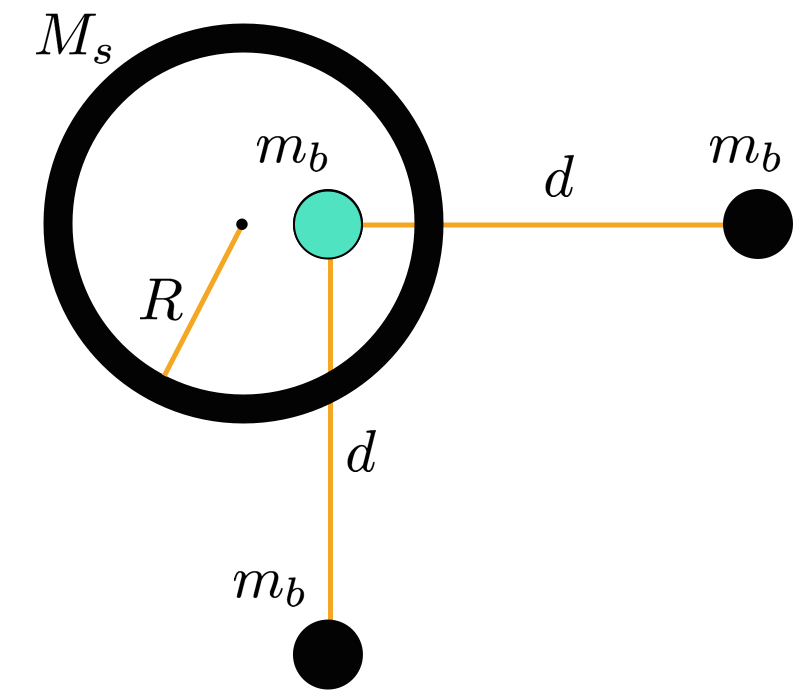# Shell it outA green sphere of mass $m_b$ is placed inside a spherical shell of radius $R$ and mass $M_s$. Two spheres identical in mass to the green sphere are placed a distance $d$ to the right and to the bottom, respectively, of it. What is the magnitude of the gravitational force on the green sphere?

×